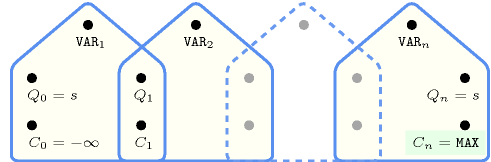## 5.249. maximum

Origin

CHIP

Constraint

$\mathrm{𝚖𝚊𝚡𝚒𝚖𝚞𝚖}\left(\mathrm{𝙼𝙰𝚇},\mathrm{𝚅𝙰𝚁𝙸𝙰𝙱𝙻𝙴𝚂}\right)$

Synonym

$\mathrm{𝚖𝚊𝚡}$.

Arguments
 $\mathrm{𝙼𝙰𝚇}$ $\mathrm{𝚍𝚟𝚊𝚛}$ $\mathrm{𝚅𝙰𝚁𝙸𝙰𝙱𝙻𝙴𝚂}$ $\mathrm{𝚌𝚘𝚕𝚕𝚎𝚌𝚝𝚒𝚘𝚗}\left(\mathrm{𝚟𝚊𝚛}-\mathrm{𝚍𝚟𝚊𝚛}\right)$
Restrictions
 $|\mathrm{𝚅𝙰𝚁𝙸𝙰𝙱𝙻𝙴𝚂}|>0$ $\mathrm{𝚛𝚎𝚚𝚞𝚒𝚛𝚎𝚍}$$\left(\mathrm{𝚅𝙰𝚁𝙸𝙰𝙱𝙻𝙴𝚂},\mathrm{𝚟𝚊𝚛}\right)$
Purpose

$\mathrm{𝙼𝙰𝚇}$ is the maximum value of the collection of domain variables $\mathrm{𝚅𝙰𝚁𝙸𝙰𝙱𝙻𝙴𝚂}$.

Example
 $\left(7,〈3,2,7,2,6〉\right)$ $\left(1,〈0,0,1,0,1〉\right)$

The first $\mathrm{𝚖𝚊𝚡𝚒𝚖𝚞𝚖}$ constraint holds since its first argument $\mathrm{𝙼𝙰𝚇}=7$ is fixed to the maximum value of the collection $〈3,2,7,2,6〉$.

Typical
 $|\mathrm{𝚅𝙰𝚁𝙸𝙰𝙱𝙻𝙴𝚂}|>1$ $\mathrm{𝚛𝚊𝚗𝚐𝚎}$$\left(\mathrm{𝚅𝙰𝚁𝙸𝙰𝙱𝙻𝙴𝚂}.\mathrm{𝚟𝚊𝚛}\right)>1$
Symmetries
• Items of $\mathrm{𝚅𝙰𝚁𝙸𝙰𝙱𝙻𝙴𝚂}$ are permutable.

• All occurrences of two distinct values of $\mathrm{𝚅𝙰𝚁𝙸𝙰𝙱𝙻𝙴𝚂}.\mathrm{𝚟𝚊𝚛}$ can be swapped.

• One and the same constant can be added to $\mathrm{𝙼𝙰𝚇}$ as well as to the $\mathrm{𝚟𝚊𝚛}$ attribute of all items of $\mathrm{𝚅𝙰𝚁𝙸𝙰𝙱𝙻𝙴𝚂}$.

Arg. properties
• Functional dependency: $\mathrm{𝙼𝙰𝚇}$ determined by $\mathrm{𝚅𝙰𝚁𝙸𝙰𝙱𝙻𝙴𝚂}$.

• Aggregate: $\mathrm{𝙼𝙰𝚇}\left(\mathrm{𝚖𝚊𝚡}\right)$, $\mathrm{𝚅𝙰𝚁𝙸𝙰𝙱𝙻𝙴𝚂}\left(\mathrm{𝚞𝚗𝚒𝚘𝚗}\right)$.

Usage

In some project scheduling problems one has to introduce dummy activities that correspond for instance to the completion time of a given set of activities. In this context one can use the $\mathrm{𝚖𝚊𝚡𝚒𝚖𝚞𝚖}$ constraint to get the maximum completion time of a set of tasks.

Remark

Note that $\mathrm{𝚖𝚊𝚡𝚒𝚖𝚞𝚖}$ is a constraint and not just a function that computes the maximum value of a collection of variables: potential values of $\mathrm{𝙼𝙰𝚇}$ influence the variables of $\mathrm{𝚅𝙰𝚁𝙸𝙰𝙱𝙻𝙴𝚂}$, and reciprocally potential values that can be assigned to variables of $\mathrm{𝚅𝙰𝚁𝙸𝙰𝙱𝙻𝙴𝚂}$ influence $\mathrm{𝙼𝙰𝚇}$.

The $\mathrm{𝚖𝚊𝚡𝚒𝚖𝚞𝚖}$ constraint is called $\mathrm{𝚖𝚊𝚡}$ in JaCoP (http://www.jacop.eu/).

Algorithm

A filtering algorithm for the $\mathrm{𝚖𝚊𝚡𝚒𝚖𝚞𝚖}$ constraint is described in [Beldiceanu01].

The $\mathrm{𝚖𝚊𝚡𝚒𝚖𝚞𝚖}$ constraint is entailed if all the following conditions hold:

1. $\mathrm{𝙼𝙰𝚇}$ is fixed.

2. At least one variable of $\mathrm{𝚅𝙰𝚁𝙸𝙰𝙱𝙻𝙴𝚂}$ is assigned value $\mathrm{𝙼𝙰𝚇}$.

3. All variables of $\mathrm{𝚅𝙰𝚁𝙸𝙰𝙱𝙻𝙴𝚂}$ have their maximum value less than or equal to value $\mathrm{𝙼𝙰𝚇}$.

Counting
 Length ($n$) 2 3 4 5 6 7 8 Solutions 9 64 625 7776 117649 2097152 43046721

Number of solutions for $\mathrm{𝚖𝚊𝚡𝚒𝚖𝚞𝚖}$: domains $0..n$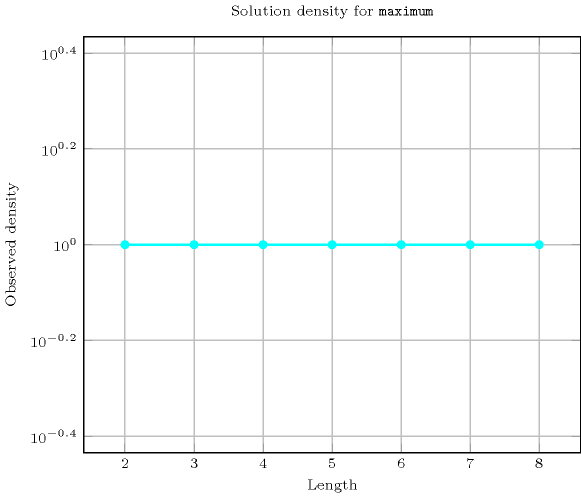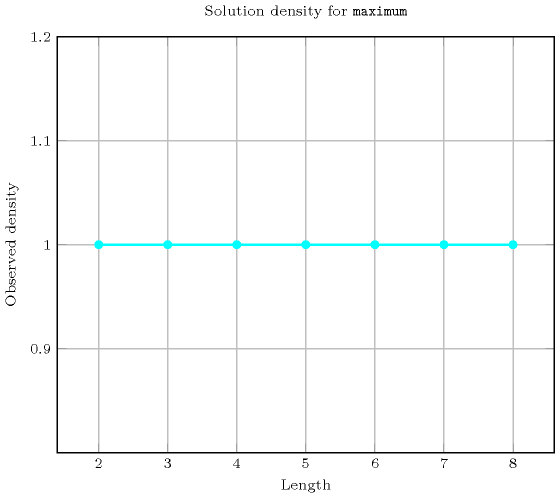Length ($n$)2345678
Total9646257776117649209715243046721
 Parameter value

01111111
137153163127255
25196521166520596305
3-3717578133671419758975
4--36921011152961741325089
5---4651310312018111288991
6----709935436074085185
7-----127360911012415
8------26269505

Solution count for $\mathrm{𝚖𝚊𝚡𝚒𝚖𝚞𝚖}$: domains $0..n$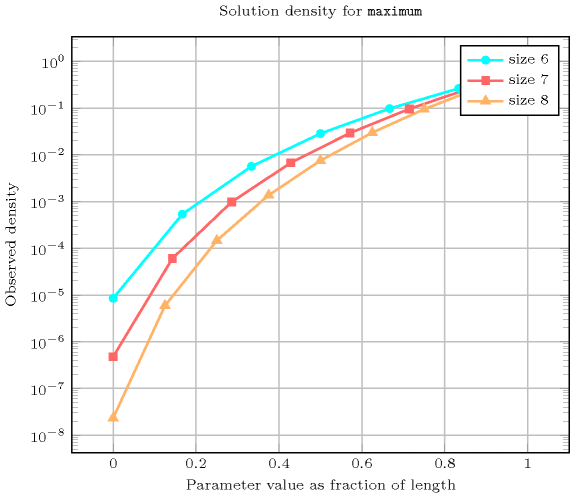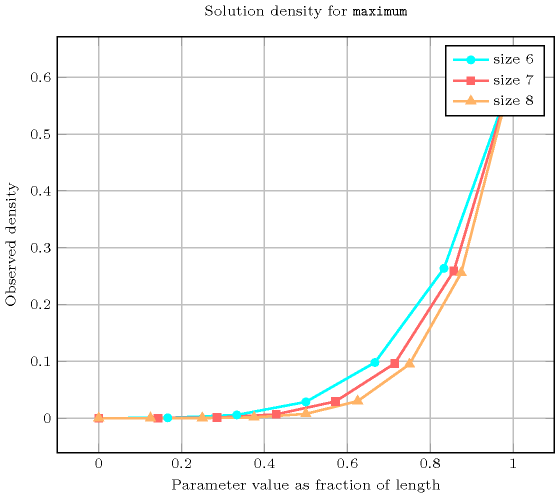Systems

max in Choco, max in Gecode, max in JaCoP, maximum in MiniZinc, maximum in SICStus.

generalisation: $\mathrm{𝚖𝚊𝚡𝚒𝚖𝚞𝚖}_\mathrm{𝚖𝚘𝚍𝚞𝚕𝚘}$ ($\mathrm{𝚟𝚊𝚛𝚒𝚊𝚋𝚕𝚎}$ replaced by $\mathrm{𝚟𝚊𝚛𝚒𝚊𝚋𝚕𝚎}\mathrm{mod}\mathrm{𝚌𝚘𝚗𝚜𝚝𝚊𝚗𝚝}$).

specialisation: $\mathrm{𝚖𝚊𝚡}_𝚗$ (maximum or order $𝚗$ replaced by absolute maximum).

Keywords
Cond. implications

$\mathrm{𝚖𝚊𝚡𝚒𝚖𝚞𝚖}\left(\mathrm{𝙼𝙰𝚇},\mathrm{𝚅𝙰𝚁𝙸𝙰𝙱𝙻𝙴𝚂}\right)$

with  $\mathrm{𝚏𝚒𝚛𝚜𝚝}\left(\mathrm{𝚅𝙰𝚁𝙸𝙰𝙱𝙻𝙴𝚂}.\mathrm{𝚟𝚊𝚛}\right)<\mathrm{𝙼𝙰𝚇}$

and   $\mathrm{𝚕𝚊𝚜𝚝}\left(\mathrm{𝚅𝙰𝚁𝙸𝙰𝙱𝙻𝙴𝚂}.\mathrm{𝚟𝚊𝚛}\right)<\mathrm{𝙼𝙰𝚇}$

implies $\mathrm{𝚑𝚒𝚐𝚑𝚎𝚜𝚝}_\mathrm{𝚙𝚎𝚊𝚔}$$\left(\mathrm{𝙷𝙴𝙸𝙶𝙷𝚃},\mathrm{𝚅𝙰𝚁𝙸𝙰𝙱𝙻𝙴𝚂}\right)$.

Arc input(s)

$\mathrm{𝚅𝙰𝚁𝙸𝙰𝙱𝙻𝙴𝚂}$

Arc generator
$\mathrm{𝐶𝐿𝐼𝑄𝑈𝐸}$$↦\mathrm{𝚌𝚘𝚕𝚕𝚎𝚌𝚝𝚒𝚘𝚗}\left(\mathrm{𝚟𝚊𝚛𝚒𝚊𝚋𝚕𝚎𝚜}\mathtt{1},\mathrm{𝚟𝚊𝚛𝚒𝚊𝚋𝚕𝚎𝚜}\mathtt{2}\right)$

Arc arity
Arc constraint(s)
$\bigvee \left(\begin{array}{c}\mathrm{𝚟𝚊𝚛𝚒𝚊𝚋𝚕𝚎𝚜}\mathtt{1}.\mathrm{𝚔𝚎𝚢}=\mathrm{𝚟𝚊𝚛𝚒𝚊𝚋𝚕𝚎𝚜}\mathtt{2}.\mathrm{𝚔𝚎𝚢},\hfill \\ \mathrm{𝚟𝚊𝚛𝚒𝚊𝚋𝚕𝚎𝚜}\mathtt{1}.\mathrm{𝚟𝚊𝚛}>\mathrm{𝚟𝚊𝚛𝚒𝚊𝚋𝚕𝚎𝚜}\mathtt{2}.\mathrm{𝚟𝚊𝚛}\hfill \end{array}\right)$
Graph property(ies)
$\mathrm{𝐎𝐑𝐃𝐄𝐑}$$\left(0,\mathrm{𝙼𝙸𝙽𝙸𝙽𝚃},\mathrm{𝚟𝚊𝚛}\right)=\mathrm{𝙼𝙰𝚇}$

Graph model

We use a similar definition that the one that was utilised for the $\mathrm{𝚖𝚒𝚗𝚒𝚖𝚞𝚖}$ constraint. Within the arc constraint, we replace the comparison operator $<$ by $>$.

Parts (A) and (B) of Figure 5.249.1 respectively show the initial and final graph associated with the first example of the Example slot. Since we use the $\mathrm{𝐎𝐑𝐃𝐄𝐑}$ graph property, the vertex of rank 0 (without considering the loops) of the final graph is outlined with a thick circle.

##### Figure 5.249.1. Initial and final graph of the $\mathrm{𝚖𝚊𝚡𝚒𝚖𝚞𝚖}$ constraint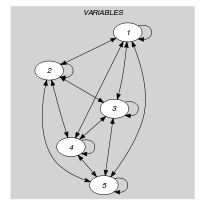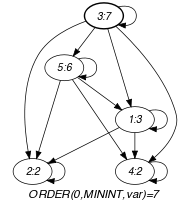(a) (b)
Automaton

Figure 5.249.2 depicts the automaton associated with the $\mathrm{𝚖𝚊𝚡𝚒𝚖𝚞𝚖}$ constraint. Let ${\mathrm{𝚅𝙰𝚁}}_{i}$ be the ${i}^{th}$ variable of the $\mathrm{𝚅𝙰𝚁𝙸𝙰𝙱𝙻𝙴𝚂}$ collection. To each pair $\left(\mathrm{𝙼𝙰𝚇},{\mathrm{𝚅𝙰𝚁}}_{i}\right)$ corresponds a signature variable ${S}_{i}$ as well as the following signature constraint: $\left(\mathrm{𝙼𝙰𝚇}>{\mathrm{𝚅𝙰𝚁}}_{i}⇔{S}_{i}=0\right)\wedge \left(\mathrm{𝙼𝙰𝚇}={\mathrm{𝚅𝙰𝚁}}_{i}⇔{S}_{i}=1\right)\wedge \left(\mathrm{𝙼𝙰𝚇}<{\mathrm{𝚅𝙰𝚁}}_{i}⇔{S}_{i}=2\right)$.

##### Figure 5.249.2. Counter free automaton of the $\mathrm{𝚖𝚊𝚡𝚒𝚖𝚞𝚖}$ constraint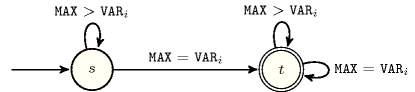##### Figure 5.249.3. Hypergraph of the reformulation corresponding to the automaton of the $\mathrm{𝚖𝚊𝚡𝚒𝚖𝚞𝚖}$ constraint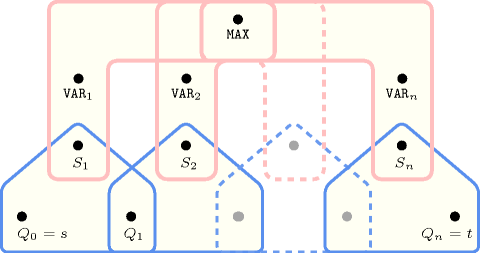Figure 5.249.3 depicts a second counter free non deterministic automaton associated with the $\mathrm{𝚖𝚊𝚡𝚒𝚖𝚞𝚖}$ constraint, where the argument $\mathrm{𝙼𝙰𝚇}$ is also part of the sequence passed to the automaton.

##### Figure 5.249.4. Counter free non deterministic automaton of the $\mathrm{𝚖𝚊𝚡𝚒𝚖𝚞𝚖}$$\left(\mathrm{𝙼𝙰𝚇},\mathrm{𝚅𝙰𝚁𝙸𝙰𝙱𝙻𝙴𝚂}\right)$ constraint assuming that the union of the domain of the variables is the set $\left\{1,2,3,4\right\}$ and that the elements of $\mathrm{𝚅𝙰𝚁𝙸𝙰𝙱𝙻𝙴𝚂}$ are first passed to the automaton followed by $\mathrm{𝙼𝙰𝚇}$ (state ${s}_{i}$ means that no value strictly greater than value $i$ was found and that value $i$ was already encountered at least once)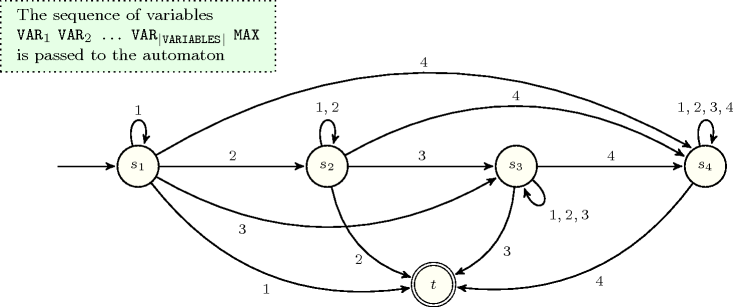##### Figure 5.249.5. Hypergraph of the reformulation corresponding to the counter free non deterministic automaton of the $\mathrm{𝚖𝚊𝚡𝚒𝚖𝚞𝚖}$ constraint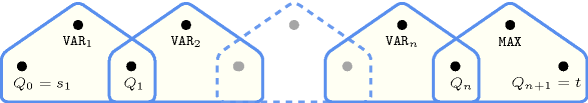Figure 5.249.6 depicts a third deterministic automaton with one counter associated with the $\mathrm{𝚖𝚊𝚡𝚒𝚖𝚞𝚖}$ constraint, where the argument $\mathrm{𝙼𝙰𝚇}$ is unified to the final value of the counter.

##### Figure 5.249.6. Automaton (with one counter) of the $\mathrm{𝚖𝚊𝚡𝚒𝚖𝚞𝚖}$ constraint and its glue constraint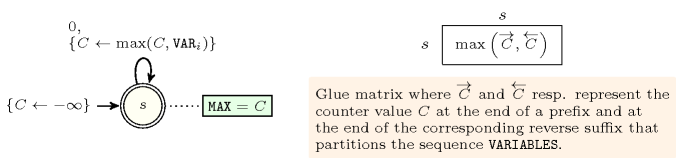##### Figure 5.249.7. Hypergraph of the reformulation corresponding to the automaton (with one counter) of the $\mathrm{𝚖𝚊𝚡𝚒𝚖𝚞𝚖}$ constraint: since all states variables ${Q}_{0},{Q}_{1},\cdots ,{Q}_{n}$ are fixed to the unique state $s$ of the automaton, the transitions constraints share only the counter variable $C$ and the constraint network is Berge-acyclic